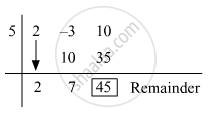Advertisement Remove all ads

# Divide Each of the Following Polynomial by Synthetic Division Method and Also by Linear Division Method. Write the Quotient and the Remainder. - Algebra

Sum
Divide each of the following polynomial by synthetic division method and also by
linear division method. Write the quotient and the remainder.
(2m^2 - 3m + 10) ÷ (m - 5)
Advertisement Remove all ads

#### Solution

Synthetic Division:

Dividend = 2m^2 - 3m + 10

Divisor = m - 5

Opposite of −5 = 5The coefficient form of the quotient is (2, 7).

∴ Quotient = 2m + 7 and Remainder = 45

Linear Method:

2m^2 - 3m + 10

= 2m(m - 5) + 10m - 3m + 10

= 2m(m - 5) + 7 (m - 5) + 35 + 10

=(m - 5) xx (2m + 7) + 45

Concept: Synthetic Division
Is there an error in this question or solution?
Advertisement Remove all ads

#### APPEARS IN

Balbharati Mathematics 1 Algebra 9th Standard Maharashtra State Board
Chapter 3 Polynomials
Practice Set 3.3 | Q 1.1 | Page 46
Advertisement Remove all ads
Advertisement Remove all ads
Share
Notifications

View all notifications

Forgot password?